Electrics Index

Alternating Current Theory

Introduction..... Nomenclature..... Generation..... Average Values & RMS values..... Vector of AC values..... Resistance in an AC circuits.....
Inductance in an AC circuits..... Capacitance in an AC circuits..... Summary of Reactance equations..... Power Factor.....
Three Phase Generation..... Mesh/ Delta Transmission..... Star Transmission..... Transformers..... Motors.....

Introduction

The notes below are of a very basic level sufficient for mechanical engineers to understand the principles involved.  For detailed information please refer to relevant reputable literature sources.  Detailed information is also available using the internet links provided at the foot of this page.

Alternating current (AC) is constantly reversing with a set frequency and in a generally sinusoidal pattern.  Virtually 100% of industrial and domestic electric power supplies are alternating current.   Most high power electrical machines and appliances are based on AC supplies.   In the UK the frequency of supply is 50 cycles /sec (Hertz).

For lighting and heating systems single phase systems are perfectly satisfactory.    When AC supplies are used for powering induction motors single phase supplies are not ideal.  Single phase induction motors are not self starting and are not very efficient without special auxiliary winding arrangements.  By using two separate windings with currents differing in phase by a quarter of a cycle or three windings with currents differing in phase by a third of a cycle, the induction motor is self starting and has better efficiency and power factor than the corresponding single phase machine.

Motors utilising two windings are called two-phase motors and motors with three windings are call three-phase motors.

These notes only discuss single phase and three phase systems.

Notation -for factors used in rotating loop equations

 A = Area of rotating loop in motor or generator (m2 ) = w.l = w.2.r B = Flux density (Tesla) e = instantaneous e.m.f. (V). E m = maximum e.m.f generated (V) f = frequency = (cycles/sec) I = current (RMS) (amperes) I m= current (maximum) (amperes) i = current (instantaneous) (amperes) l = length of rotating loop (m) r = radius of rotating loop (m) w = width of rotating loop (m) = 2.r n = speed of rotation of loop (revs/s) u = velocity of sides of rotating loop (m/s) V = Potential difference (RMS) (volts) V m= Potential difference (maximum) (volts) v = voltage (instantaneous) (volts) N = Number of windings ω = Angular velocity of loop (rads/sec) = 2.π.f

Generation of Single Phase e.m.f

At the most basic level a simple electrical generator as shown in principle below provides a sinusoidal voltage output.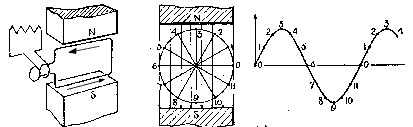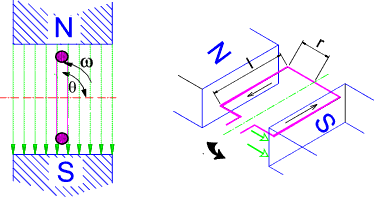The e.m.f generated in one side on the loop is B.l.u. sin θ ..(Zero voltage results when the side of the loop is moving parallel to the lines of magnetic flux and voltage = B.l.u when the velocity (u) is normal to the lines of flux.) .    ( ref Electromagnetic Induction )

The total emf generated by one side of the loop moving past the N pole + the other side of the loop moving past the S pole = 2.B.l.u sin .θ.

The velocity u of the sides of the loop is ω.r and therefore the e.m.f. generated by the rotating loop at any angle=

e = 2.B.l.u.sin θ = 2.B.l.ω.r.sin θ = B.A.ω.sin θ = B.A.2.π.n.sin θ

Note: A = area of covered by the loop = l.2.r

Alternatively if E m =B.A.ω the instantaneous e.m.f. =

e    =   E m sin θ    =   E m sin ω.t

If the loop is replaced by a coil of N turns in series each having a area (2.r.l) = A then the maximum e.m.f. generated in the coil =
E m = B.A.2.π .n.N volts.   The instantaneous e.m.f at any angle of rotation =

e = E m sin θ = B.A.2.π .n.N sin θ

Average and R.M.S Values of sinusoidal

These notes relate primarily to cyclic variations of a variable.   The principles also apply to variables which are constantly changing over time.

There is a requirement to provide a single practical value to identify the magnitude of the variable (current, voltage etc) which varies in magnitude.   Two methods of quantifying a representive value are available : "The Average Value" and "The Root Mean Square Value."

The Average Value
Intuitively the average value of the current or the voltage is the one which provides a useful practical measure of its magnitude.  When an a.c. variable has been rectified the unidirecional current will deflect a d.c. meter.  If the meter is has a suitable movement the meter will show the average value of the current.    Three waveforms are shown below and the average value of their full wave rectified values are indicated.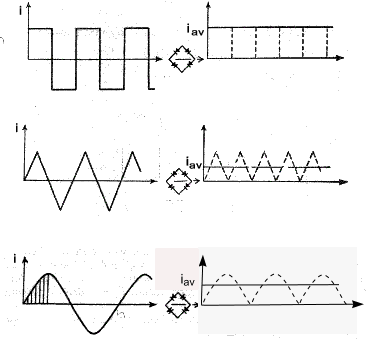For the square wave pattern the average value of the full wave rectified form is i av = I m
For the triangular wage pattern the average value of the full wave rectified form is I av = I m /2.
For the sinusoidal wage pattern the average value of the full wave rectified form is I av = 0,637 I m
Note: For all of these forms the average value for the half wave rectified version, when the negative part of the cycle is cut off , is half the value as shown above..

The Root Mean Square Value

In practical electrical engineering the average value is of little importance.    It is most important to consider the work that the electricity can do either in heating or in mechanical work.  This is generally related to the power equivalent of the current W = I2 /R or V2 /R

Consider one cycle of the square waveform as shown below. (two cycles actually shown)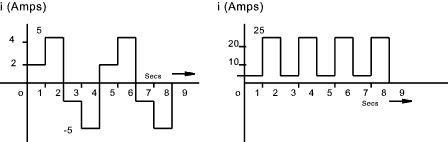Assuming the current is delivering energy by heating a 4 ohm resistor.   The total energy delivered over a complete cycle =

Energy = Σ i 2 R.t = [22.1 + 52.1 + -22.1 + -52.1].4 =58.4 =232 Joules

The equivalent direct current to produce the same heating effect over a complete cycle = I.
This value of I called the Root Mean Square Value =

I 2 R.t= =232 Joules     Therefore   I = Sqrt (232 / R.t) = Sqrt [ 232 /( 4.4)] = 3.807 Amperes

Note: The average full wave rectified value = (2.1+5*1)*2/4 = 3.5 Amperes

For a sinusoidal current The RMS value for the current (I)

I = 0,707.Im

 1) Average value for a sinusoidal variable Consider a Sinusoidal current i = Im sin θ over a half Cycle...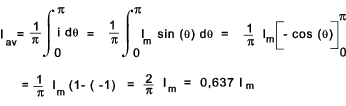2) Root Mean Square value for a sinusoidal variable Consider a Sinusoidal current i = Im sin θ.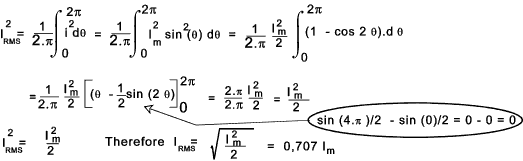Form Factor

The form factor for a sin wave is defined as the R.M.S value /Average value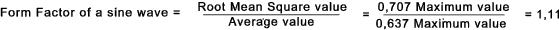Peak Value

The peak or crest value of a sine wave is defined as the maximum value /the R.M.S value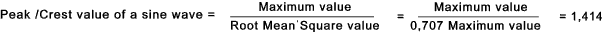Vector Indication of AC values

A sinusoidal variable can be shown as a plot of amplitude against time or   θ = ω.t
In can also be shown as a rotating vector or phaser. Both these methods are illustrated below.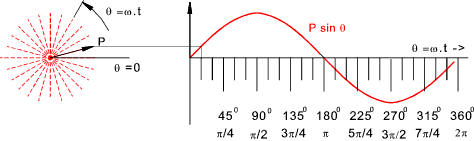The rotating vector on the left is assumed to be rotating at a constant angular velocity ω
Its projection on the vertical axis = P sin ω t. = P.sin θ is plotted as shown on the right

Two sinusoidal alternating variables with different amplitudes and phases are shown together as below.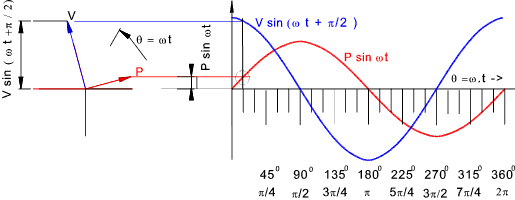The two vectors can be added as shown below to obtain a resultant vector R.sin (ω t + z).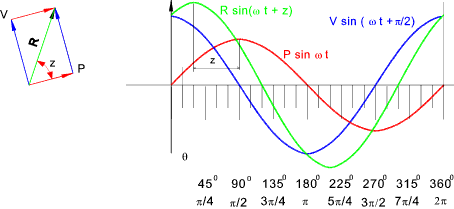R and z are easily calculated as below as shown..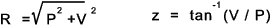The methods of showing and adding sinusoidal variables is used when manipulating electrical currents currents flowing in circuits which include resistances, inductances, and capacitances

Resistance In an AC Circuit

Considering a AC circuit with a resistance of R.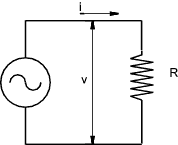The ratio of the potential difference (V) across a resistor to the current (I) flowing through it it is a constant - This is Ohms law .  This applies to steady currents and to varying currents.  For the case of an Alternating current the potential difference and the current are in phase and the current at any time =

i = v /R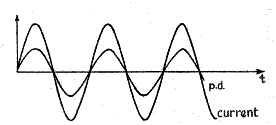Inductances In an AC Circuit

Inductance (L) is measured using the unit of 1 henry which is the inductance in when 1 volt is produced by a current changing at the rate of 1 ampere/second.   If there is a steady DC voltage across an inductance it will result in no induced e.m.f and the only effect limiting the steady current will be the associated resistance.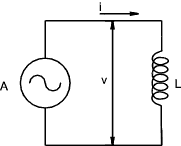Consider a sine wave current through an inductance of L henries represented by the equation.. i = Im sin ωt.    (t being the time in second after the current has passed through zero from negative to positive.)  The instantaneous value of the induced e.m.f resulting from this changing current =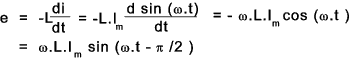The amplitude of the induced e.m.f = ω.L.Im and the resulting e.m.f sine wave effectively lags the current by ω /2 .   The sine waves are shown below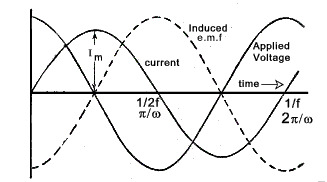Note: The frequency f = ω /(2.π) and the wavelength = 1/f = 2.π /ω

Assuming there is no resistance all of the applied voltage is absorbed in neutralising the induced e.m.f . Therefore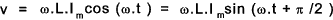The applied voltage leads the current by π /2 and the maximum applied voltage = ω.L.I m that is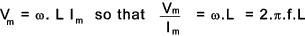The inductive reactance is expressed as ohms and is represented by the symbol X L.   Therefore I = V /X L = V /(2.π.f.L).   Unlike a resistance there is no energy absorbed in an inductance subject to a sine wave applied voltage.

Capacitances In an AC Circuit

The Capacitance C in which 1 coulomb is stored for a potential difference of 1 volt is a Farad

The charge on a capacitor Q = C.V and therefore dQ/dt = C dV/dt and therefore i = = C dV/dt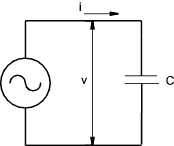Referring to the curve below at time = 0 the rate of increase of applied voltage is such that the current is at a maximum value.
In the period from t = 0 to t = π /2. The rate of change of the applied voltage reduces in a sinusoidal wave form to zero resulting in a fall of current flow to zero.  The curve illustrates the relationship of the current to the applied voltage throughout the cycle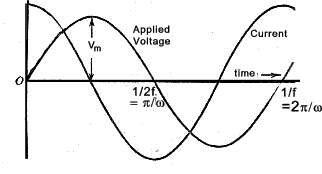Note: The frequency f = ω /(2.π) and the wavelength = 1/f = 2.π /ω

Considering sinusoidal voltage applied across a purely capacitive circuit the following relationships apply.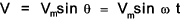As the instantaneous value of the current is i = C.dv/dt then..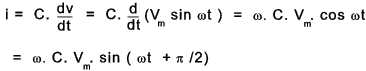It is clear that the current leads the voltage by π /2 and the maximum value = ωC Vm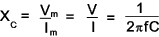Summary of AC Circuit formula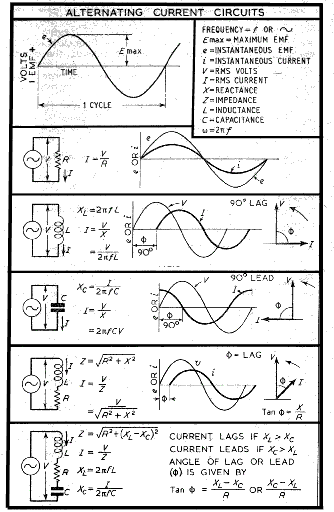Power Factor

Consider a conductor with applied AC voltage with an out of phase current.  The curve below shows an applied voltage with a current which is lagging the voltage by φ.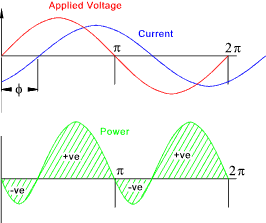The instantaneous voltage v = V m sin(ω.t )
The instantaneous current i = I m sin(ω.t - φ )
The instantaneous power p = i.v = V m sin(ω.t ).I m sin(ω.t - φ )
= 0,5.V mI m.[cos φ - cos (2ω - φ )]
= 0,5.V mI m.(cos φ)   -   0,5.V mI m.cos (2ω - φ )

Over a complete cycle the average value of cos (2ω - φ ) = 0. ......(it is varying sinusoidally )
The average value of power over one cycle is therefore. 0,5.V mImx.(cos φ).......( cos φ is the phase difference- a fixed value)
The average power =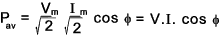....V & I are RMS values

It is clear that if the phase difference is +/- (π /2) a purely capacitive or inductive load then the power from the alternator = 0.
If the phase difference is 0 Then the power from the alternator is V. I.

The product of the RMS voltage and current (V.I) is the volt-amperes which is not the same as V.I. cos φ which is the power expressed in watts.   The factor cos φ is known as the power factor and is an important factor in power distribution systems.

Power in watts = Voltamperes .power factor = V.I.cos φ
Power factor (cos φ) = power (watts) / V.I
=   I2 R / V.I    =   I R / V    =   IR / I.Z    =    R / Z
Z = impedance of circuit.

For an alternative view on the topic consider an alternating voltage V with a current lagging by φ shown vectorially below..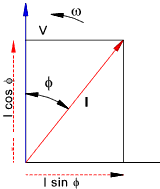The current can be resolved into two components I cos φin line with the voltage and I sinφ at right angles to the voltage.  The power due to the first component is VI cosφ is the power or active component.  The power due to the second component = V (I.sinφ) cos 900 = 0 this is the reactive or wattless component of the current

Generation of Three Phase supplies

Consider three loops each position 120o relative to each other rotating in a magnetic field as shown below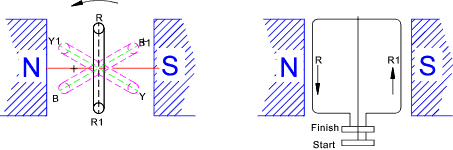The generated e.m.f's are shown below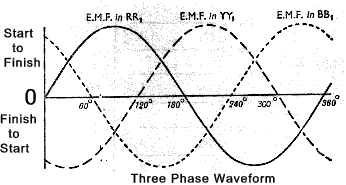The loops are being rotated anti-clockwise and each loop is producing exactly the same emf with the same amplitude and frequency but the loop Y Lags loop R by 1200 and the loop B lags loop Y by 1200.    This is the same for the associated loop Y 1, B 1, and R 1 . At any moment the e.m.f generated in the three loops are as follows

e R = E m sin ( θ )
e Y = E m sin ( θ - 1200)
e B = E m sin ( θ - 2400 )

Mesh /Delta Connection of Three Phase supplies

The three phases can be shown as below as separate circuits with six conductors between the generator loops and the respective loads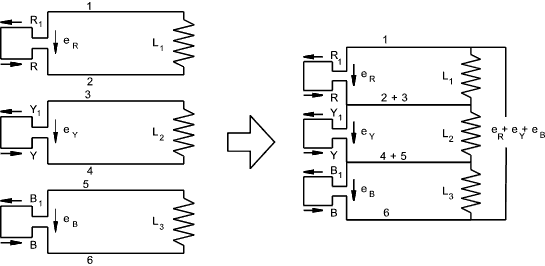This system results in six conductors and can be simplified to reduce the number of conductors.  Initially it is possible to replace conductors 2 and 3 with one conductor and conductors 4 and 5 with one conductor.   It can also easily be proved that there is no voltage difference between 6 and 1 allowing these also to be connected as one conductor and any stage in the cycle, as follows

e R + e Y + e B = E m [sin ( θ ) + sin ( θ -1200 ) + sin ( θ -2400 ) ]

= E m [ sin ( θ ) + sin θ.cos 1200 - cos θ.sin 1200 + sin θ.cos 2400 - cos θ.sin 2400 ]

= E m [ sin ( θ ) - 0,5.sin θ - 0,866. cos θ - 0,5 .sin θ + 0,866. cos θ ]
= 0

This method of connecting the conductors in a three-phase system is called a Mesh or Delta connection and is generally shown as below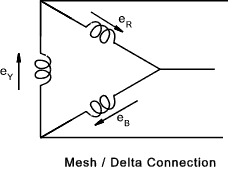Star Connection of Three Phase supplies

If the three start conductors 1,3,and 5 connected as one conductor NM as shown (in blue) below then the current flow is as shown...Three phase motors can be connected to the line conductors R.Y and B while lights, heaters etc are usually connected between the line conductors and the neutral line as shown by (L 1,L 2 & L 3 )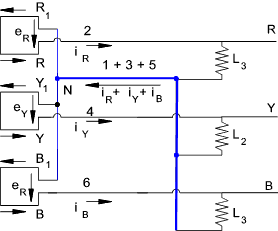..

This conductor arrangement is normally shown as below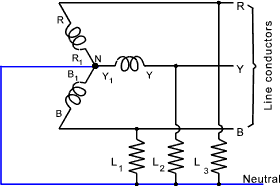Ideally if the loads L 1, L 2 and L 3 are the same then the phase currents have the same peak value I m and differ in phase by 1200

i 1 = I m sin ( θ )
i 2 = I m sin ( θ -1200)
i 3 = I m sin ( θ -2400 )

The resultant total current in the Neutral line = i 1 +i 2 +i 3

= I m[sin (θ) + sin ( θ -1200 ) + sin ( θ -2400 ] = I m. (0) = 0

Hence with balanced loads there is no load in the neutral line at any instant and the neutral line can be removed resulting in the three wire star system as shown below...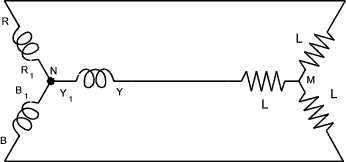It can be proved that at every instant, for balanced loads the algebraic sum of the currents flowing in the three conductors is zero

Transformers

A transformer is an electromagnetic device having two or more stationary coils coupled through a mutual magnetic flux.   Transformer are used to increase or decrease voltages.  Tranformers are essential in the electrical power transmission industry allowing power to be generated at one voltage and then stepped up to higher voltages for long distance transmission.  The voltages can then be stepped down for driving motors and operating heaters and lamps.

The most efficient use of electrical power is achieved by generating the power at one voltage then increasing the voltage to very high values for long distances and then reducing the voltage to lower values for safe use in industry and in the home.  A very crude single line representation of the 3 phase transmission system in the UK is shown below.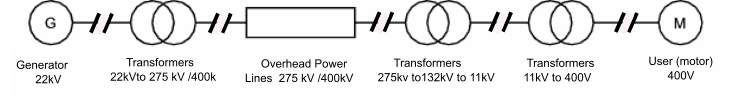In the UK for long distance power transmission the voltage is stepped up to 400/275 kV.   It is then progressively reduced to 11kV for transmission within urban areas.    It is locally progressively reduced to 380-415V (phase to phase -3 phase) 220-240 Volts (phase to nuetral - single phase)

More detailed notes on the UK Power supply and distribution sytems are found a t UK Electical Power Industry.

Note:..The new "harmonised voltage limits" in Europe are now:230V -10% +6% (i.e. 207.0 V-243.8 V) in most of Europe (the former 220V nominal countries), and 230V -6% +10% (i.e. 216.2 V - 253.0 V) in UK (former 240V nominal).

Principle of Action of a transformer

A transformer consists of an metal core of laminated sheets about 0,35mm thick insulated from each other to reduce eddy current losses.  The vertical parts as shown are called the limbs and the horizontal parts are called the yokes.   The two coils are wound on the limbs.  One coil is called the primary coil and the other is called the secondary coil.

The metal core is made from a ferromagnetic metal selected because of its magnetic properties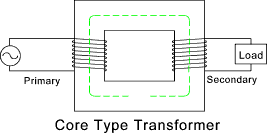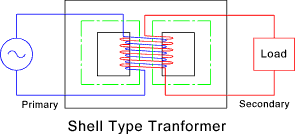An alternating voltage applied to the primary coil results in an alternating current which produces an alternating magnetic flux in the metal core.   The current /flux level is just sufficient to induce an e.m.f that is practically equal and opposite to the applied voltage.   The mean path of the alternating flux is indicated in the figure.   Assuming all of the flux passes through the primary and the secondary winding,it induces the same e.m.f. in each turn of both coils.   Hence if N1 and N2 are the number of turns in the primary and secondary coils respectively .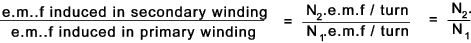When the secondary winding is in open circuit its terminal voltage is the same as the induced e.m.f .    The primary current is such that the induced e.m.f is equal and opposite to the applied alternating voltage.

When a load is connected to the secondary winding a current flows.  This secondary current induces a magnetic flux which tends to reduce the current flow (Lenz's Law).   The induced flux tends to reduce the magnetic flux and the resulting back back e.m.f.in the primary windigs    This changes the difference between the applied voltage and the back e.m.f.in the primary windings from (say 0,05% to 1%).    As a result the primary current increases, by say 20 times the no-load current.  The demagnetising ampere turns of the secondary winding are thus neutralised by the increase in the primary ampere turns..   As the initial no-load primary ampere-turns are very small it can be stated that

I1.N1 = I2.N2 ..therefore I1/I2= N2 /N1 = V2 /V1

In practice the above are all approximate

Transformer Losses

The energy wasted in a transformer can be divided into magnetic leakage losses copper losses and iron losses.   The iron losses are hysteresis losses and eddy current losses.   The hysterisis losses are described on webpage Electromagnetism - Hysteresis Losses.

Magnetic leakage occurs because all of the flux established by the current in the primary coil does not link with the secondary and all of the flux due to the current induced in the secondary coil does not link with the primary coil. The effect of this is to produce a slightly lower secondary voltage than that calculated by the relevant formula.  In commercial transformers there is virtually zero magnetic losses.

The copper losses are simply the normal losses to heat as a result of the resistance to of the wires making up the primary and secondary windings.  These losses are related to I2 R and therefore increase with the power transferred by the transformer.   The copper power losses are simply the sum of the I2.R of each winding.

The eddy current losses are caused by induced currents circulating in the transformer core and, to a small extent, in the copper windings.  If the core were solid then the induced current would be significant because there would be very low resistance.  By making the core from thin laminated steel plates separated by insulation the conduction path is reduced such that the eddy current losses are minimised.  The effect of these losses is to reduce the secondary voltage below that obtained by the relevant formula.  Refer to figure below..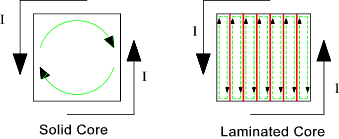Motors

There are a number of methods of classifying AC motors

1) Single Phase and Three phase.. Most AC motors are three phase.
2) Motors with shunt characteristics relating to torque and speed and motors with series type characteristics and motors running at constant speed (synchronous speed)
3) Motors in which all of the current is supplied from outside and motors in which the current in one part (generally the rotor) are produced by induction.

Single phase motors with series characteristics are used primarily for fraction kW motors used for vacuum cleaners , small tools etc.   Single phase induction motors are only self starting if special provisions are provided to drive them up to synchronous speed
The vast majority of motors are polyphase induction motors.  An induction motor consists of a rotor and a stator.   For notes on AC inductance motors ref to webpage AC Motors

A synchronous motor is basically an alternator which is brought up to a synchronous speed and then connected to an external polyphase supply.  It will then run as a motor at constant speed.  Synchronous motors are not self starting and they require a source of direct current (usually an exciter on the motor shaft) to provide the exciting field on the rotor.  Synchronous motors will, if over excited run at a low leading power factor which will compensate for the low lagging power of other loads such as induction motors.   Synchronous motors are used in industry to compensate for the lagging power factors associated with induction motors providing a higher overall power factor.  Synchronous motors are sometimes used when a long term constant speed at load is required with infrequent starts

 Useful relevant Links School for champions Alternating Current...Easy starter site All about Circuits Vol 2 AC... Very clear and easy to follow document Hyperphysics- AC Circuits... Excellent reference site for all Physics subjects Integrated Publishing - Concepts of alternating current ...Useful Notes Lessons in Electric Circuits -Vol 2 Alternating Currents ...Free on-line book -great

Electrics Index